# Boundary Value Problems

Also found in: Acronyms.
The following article is from The Great Soviet Encyclopedia (1979). It might be outdated or ideologically biased.

## Boundary Value Problems

problems in which it is required to find, from among a class of functions defined on a given region, that function that satisfies specified conditions on the boundary of the region.

Functions that describe actual natural (physical, chemical, and other) phenomena are as a rule solutions of equations of mathematical physics that are derived from the general laws these phenomena obey. When these equations possess whole families of solutions, boundary or initial conditions are specified additionally to permit a particular solution to be uniquely selected. Whereas boundary conditions are specified exclusively at the boundary points of the region in which the solution is found, initial conditions may be specified on a fixed set of points within the region. For example, the equationhas an infinite set of solutions u(x1, x2) = f(x1 + x2) + f1(x1x2) where f and f1 are arbitrary twice continuously differentiable functions. However, within a rectangle –ax2a, 0 ≤ x1l, in a plane with rectangular Cartesian coordinates x1, x2, equation (1) has a unique solution u(x1, x2) that satisfies the boundary conditions

(2)u(0, x2) = 0 u(l, x2) = 0 −ax2 ≤ a

and the initial conditions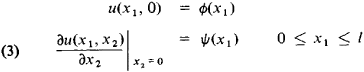In this case, the twice continuously differentiable functions ϕ and ψ are considered to be preassigned. If the variable x2 is time t, then the solution u(x, t) of equation (1) that satisfies conditions (2) and (3) describes the oscillation of an elastic string of length l whose ends are fastened to the points (0, 0) and (0, l). The problem of finding the solution to equation (1) given the conditions (2) and (3) is the simplest example of what is known as a mixed boundary value problem.

In general, boundary value problems are problems in which a solution u(x) = u(x1 . . . , xn) of the equation

Du(x) = 0 xG

is sought in a given region G of a space of independent variables (x1, . . . , xn) = x under the requirement that the desired function u(x) satisfy on the boundary S of region G the boundary condition

Bu(x) = 0 yS

where D and B are the given operators. Moreover, D as a rule is a differential or an integro-differential operator. The boundary S is called the support of the boundary data (5).

When the operators D and B are linear, the boundary value problem (4), (5) is called a linear boundary value problem. Under the assumptions that S is an (n − 1)-dimensional hypersurface and that D is a second-order linear differential operator,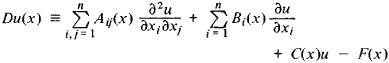and

Bu(y) = u(y) − f(y)

where Aij, Bij, C, F, and f are given functions, the problem (4),

(5) is called the first boundary value, or Dirichlet, problem. But if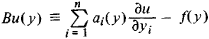where ai, i = 1, . . . ,n, and f are given functions, it is called the oblique derivative problem. In particular, when the vector (a1 . . . ,an) coincides with the conormal to S, the oblique derivative problem is called the second boundary value, or Neumann, problem. The Dirichlet (Neumann) problem is said to be homogeneous if

F(x) = 0 F(y) = 0

The Dirichlet and Neumann problems have been studied thoroughly in bounded regions possessing sufficiently smooth boundaries for a real operator D that is uniformly elliptic, that is, under the conditions that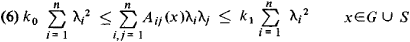where λ1, . . . ,λn are arbitrary real parameters and k0 and k1 are fixed nonzero numbers of the same sign.

The following statements are valid if the coefficients of D and B are sufficiently smooth and if D is uniformly elliptic. (1) The number k of linearly independent solutions of the homogeneous Dirichlet (Neumann) problem is finite. (2) For the Dirichlet (Neumann) problem to be solvable, it is necessary and sufficient that the functions F(x) and f(y) obey k additional restrictions that are of the type of orthogonality conditions. (3) When the condition

C(x) ≤ 0 x∊G

is observed, the Dirichlet problem always has a solution, moreover, a unique solution. (4) The Dirichlet problem always has a solution (a unique solution) in a region G whose diameter is sufficiently small. (5) A small variation of the boundary data when the Dirichlet (Neumann) problem is uniquely solvable induces a small variation in the solution (that is, the solution is stable).

When D is the Laplace operator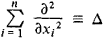the solution of the Dirichlet problem in a bounded region possessing a sufficiently smooth boundary always exists and is unique. Moreover, for certain special kinds of regions it can be written in explicit form. Thus, for example, when n = 1, over the interval −1 < x < 1 this solution has the form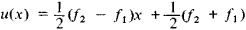where f1 = u(−1) and f2 = u(1); when n = 2 and n = 3, in the circle ǀxǀ < 1 and the sphere ǀxǀ < 1, respectively,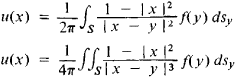where ǀx − yǀ is the distance between the points x and y. A linear boundary value problem is called a Fredholm problem if the statements (1) - (5) are valid for it.

In boundary value problems for elliptic equations, it is usually assumed that the support of the boundary condition is the entire boundary S of the region G.

If condition (6) of uniform ellipticity is not satisfied but the operator D is elliptic in the sense that the quadratic form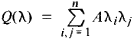is positive (or negative) in region D, then a certain part of the boundary S of region G may have to be freed of boundary data in order to preserve the Fredholm character of the boundary value problem.

A linear boundary value problem is not, generally speaking, a Fredholm problem even under the requirement of uniform ellipticity of the differential operator D. In particular, the oblique derivative problem may turn out not to be a Fredholm problem if the vector (a1 . . . , an) at certain points of S lies in a plane tangent to S.

When the differential operator D is not elliptic, the boundary value problem (4), (5) may have no meaningful sense at all if part of the boundary S of region G is not freed of boundary data and if no definite (occasionally extremely strong) restrictions are placed on the structure of the support of the boundary data. For example, the heat equation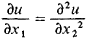which is a typical example of a parabolic equation, has a unique solution u(x1, x2) in the square bounded by the lines x1 = 0, x1 = 1, x2 = 0, and x2 = 1 that satisfies the boundary conditions

u(0, x2) = f(x2) 0 ≤ x2 ≤ 1

u(x1, 0) = ϕ(x1) 0 ≤ x1 ≤ 1

u(1, x2) = ψ(x2) 0 ≤ x2 ≤ 1

f(0) = ϕ(0) ψ(0) = ϕ(1)

for arbitrary sufficiently smooth data f, ϕ, and ψ. Consequently, the boundary condition u(x1, 1) = θ(x1) on the interval 0 ≤ x1 ≤ 1, can no longer be specified arbitrarily. In precisely the same fashion the simplest hyperbolic-type equation (1) examined above in the square bounded by the lines x1 + x2 = 0, x1x2 = 0, x1 + x2 = 1, x1x2 = −1 has a unique solution u(x1, x2) satisfying the boundary conditions

u(x1, x1) = f(x1) 0 ≤ x1 ≤ 1/2

u(x1x1) = ϕ(x1) −1/2 ≤ x1 ≤ 0

f(0) = ?(0)

for arbitrary sufficiently smooth data f and ϕ. It is evident that in this case the boundary values u(x1, 1 + x1), on the interval 1/2 ≤ x1 ≤ 0, and u(x1, 1 − x1), on the interval 0 ≤ x1 ≤ ½, likewise cannot be prescribed arbitrarily.

Boundary value problems must be posed in a special way when the differential operator D is not of the same type (elliptic, hyperbolic, parabolic) in different parts of the region G, that is, when equation (4) is of mixed type.

Integral equation methods (potential theory), a priori estimates, and finite differences are widely used in studying boundary value problems.

### REFERENCES

Bernshtein, S. N. Sobr. soch., vol. 3. Moscow, 1960.
Vekua, I. N. Novye metody resheniia ellipticheskikh uravnenii. Moscow-Leningrad, 1948.
Vladimirov, V. S. Uravneniia matematicheskoi fiziki. Moscow, 1967.
Muskhelishvili, N. I. Singuliarnye integral’nye uravneniia, 3rd ed. Moscow, 1968.
Petrovskii, I. G. Lektsii ob uravneniiakh s chastnymi proizvodnymi, 3rd ed. Moscow, 1961.
Sobolev, S. L. Nekotorye primeneniia funktsial’nogo analiza v matematicheskoi fizike. Novosibirsk, 1962.
Tikhonov, A. N., and A. A. Samarskii. Uravneniia matematicheskoi fiziki, 3rd ed. Moscow, 1966.

References in periodicals archive ?
Existence results for a class of nth order m-point boundary value problems in Banach spaces.
The Boundary Value Problem in the Convex and Convex-Concave Case with 0 < [beta] < 1
Cui, "Positive solutions for boundary value problems of fractional differential equation with integral boundary conditions," Journal of Function Spaces, vol.
Purnaras, "Existence results for nonlocal boundary value problems of nonlinear fractional q-difference equations Difference equations: new trends and applications in biology, medicine and biotechnology," Advances in Difference Equations, vol.
JEFFREY, An efficient analytical approach for solving fourth order boundary value problems, Comput.
Ozbilge, "Numerical solution and distinguishability in time fractional parabolic equation," Boundary Value Problems, vol.
Consider the process of solving boundaryvalue problems using RBFNs using an example of the boundary value problem, given in the following operator form:
Jankowski, Multipoint boundary value problems for ODEs.
Xu, "Positive solutions to fractional boundary value problems with nonlinear boundary conditions," Boundary Value Problems, 2014:225, 15 pages, 2014.
Exact solution of the boundary value problem of bending bandpass shallow shell, which is supported by intermediate thin semi-infinite rib, type Winkler foundation was obtained in ; and supported by intermediate thin semi-infinite rigid support, was obtained in .

Site: Follow: Share:
Open / Close